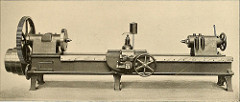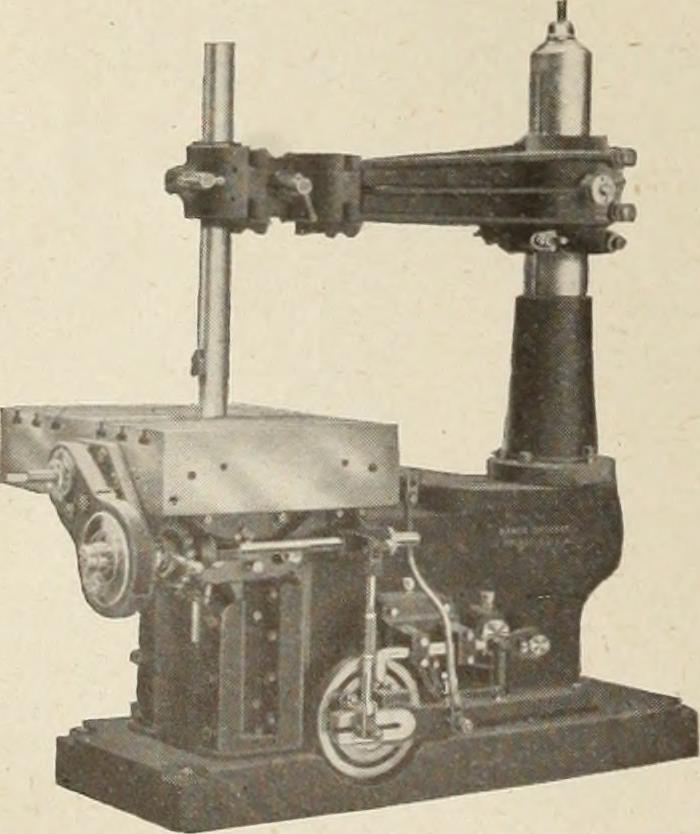#5 Reasons Rate of Change is Important

A simplifying assumption associated with using MTTF or MTBF implies a constant hazard rate. Some assume we’re in the useful life section of the bathtub curve. Others do not understand what assumptions they are making.

Using MTTF or MTBF has many problems and as regular reader here know, we should avoid using these metrics.

By using MTTF or MTBF we also lose information. We are unable to measure or track the rate of change of our equipment or system’s failure rates (hazard rate). The simple average is just an average and does not contain the essential information we need to make decisions.

Let’s explore five different reasons the rate of change of a failure rate is important to measure and track. Continue reading 5 Reasons Rate of Change is Important

#Are the Measures Failure Rate and Probability of Failure Different?

Failure rate and probability are similar. They are slightly different, too.

One of the problems with reliability engineering is so many terms and concepts are not commonly understood.

Reliability, for example, is commonly defined as dependable, trustworthy, as in you can count on him to bring the bagels. Whereas, reliability engineers define reliability as the probability of successful operation/function within in a specific environment over a defined duration.

The same for failure rate and probability of failure. We often have specific data-driven or business-related goals behind the terms. Others do not.
If we do not state over which time period either term applies, that is left to the imagination of the listener. Which is rarely good.

## Failure Rate Definition

There at least two failure rates that we may encounter: the instantaneous failure rate and the average failure rate. The trouble starts when you ask for and are asked about an item’s failure rate. Which failure rate are you both talking about?

The instantaneous failure rate is also known as the hazard rate h(t)

￼￼￼￼$\displaystyle h\left( t \right)=\frac{f\left( t \right)}{R\left( t \right)}$

Where f(t) is the probability density function and R(t) is the relaibilit function with is one minus the cumulative distribution function. The hazard rate, failure rate, or instantaneous failure rate is the failures per unit time when the time interval is very small at some point in time, t. Thus, if a unit is operating for a year, this calculation would provide the chance of failure in the next instant of time.

This is not useful for the calculation of the number of failures over that year, only the chance of a failure in the next moment.

The probability density function provides the fraction failure over an interval of time. As with a count of failures per month, a histogram of the count of failure per month would roughly describe a PDF, or f(t). The curve described for each point in time traces the value of the individual points in time instantaneous failure rate.

Sometimes, we are interested in the average failure rate, AFR. Where the AFR over a time interval, t1 to t2, is found by integrating the instantaneous failure rate over the interval and divide by t2 – t1. When we set t1 to 0, we have$\displaystyle AFR\left( T \right)=\frac{H\left( T \right)}{T}=\frac{-\ln R\left( T \right)}{T}$

Where H(T) is the integral of the hazard rate, h(t) from time zero to time T,
T is the time of interest which define a time period from zero to T,
And, R(T) is the reliability function or probability of successful operation from time zero to T.

A very common understanding of the rate of failure is the calculation of the count of failures over some time period divided by the number of hours of operation. This results in the fraction expected to fail on average per hour. I’m not sure which definition of failure rate above this fits, and yet find this is how most think of failure rate.

If we have 1,000 resistors that each operate for 1,000 hours, and then a failure occurs, we have 1 / (1,000 x 1,000 ) = 0.000001 failures per hour.

Let’s save the discussion about the many ways to report failure rates, AFR (two methods, at least), FIT, PPM/K, etc.

## Probability of Failure Definition

I thought the definition of failure rate would be straightforward until I went looking for a definition. It is with trepidation that I start this section on the probability of failure definition.

To my surprise it is actually rather simple, the common definition both in common use and mathematically are the same. There are two equivalent ways to phrase the definition:

1. The probability or chance that a unit drawn at random from the population will fail by time t.
2. The proportion or fraction of all units in the population that fail by time t.

We can talk about individual items or all of them concerning the probability of failure. If we have a 1 in 100 chance of failure over a year, then that means we have about a 1% chance that the unit we’re using will fail before the end of the year. Or it means if we have 100 units placed into operation, we would expect one of them to fail by the end of the year.

The probability of failure for a segment of time is defined by the cumulative distribution function or CDF.

## When to Use Failure Rate or Probability of Failure

This depends on the situation. Are you talking about the chance to failure in the next instant or the chance of failing over a time interval? Use failure rate for the former, and probability of failure for the latter.

In either case, be clear with your audience which definition (and assumptions) you are using. If you know of other failure rate or probability of failure definition, or if you know of a great way to keep all these definitions clearly sorted, please leave a comment below.

#What if all failures occurred truly randomly?

The math would be easier.

The exponential distribution would be the only time to failure distribution. We wouldn’t need Weibull or other complex multi parameter models. Knowing the failure rate for an hour would be all we would need to know, over any time frame.

Sample size and test planning would be simpler. Just run the samples at hand long enough to accumulated enough hours to provide a reasonable estimate for the failure rate.

## Would the Design Process Change?

Yes, I suppose it would. The effects of early life and wear out would not exist. Once a product is placed into service the chance to fail the first hour would be the same as any hour of it’s operation. It would fail eventually and the chance of failing before a year would solely depend on the chance of failure per hour.

A higher failure rate would suggest it would have a lower chance of surviving very long. Although it could still fail in the first hour of use as if it had survived for one million hours and then it’s chance to fail the next hour would still be the same.

## Would Warranty Make Sense?

Since by design we cannot create a product with a low initial failure rate we would only focus on the overall failure rate. Or the chance of failing over any hour, the first hour being convenient and easy to test, yet still meaningful. Any single failure in a customer’s hands could occur at any time and would not alone suggest the failure rate has changed.

Maybe a warranty would make sense based customer satisfaction. We could estimate the number of failures over a time period and set aside funds for warranty expenses. I suppose it would place a burden on the design team to create products with a lower failure rate per hour. Maybe warranty would still make sense.

If there are no wear out mechanisms (this is a make believe world) changing the oil in your car would not make any economic sense. The existing oil has the same chance of engine seize failure as any new oil. The lubricant doesn’t breakdown. Seals do not leak. Metal on metal movement doesn’t cause damaging heat or abrasion.

You may have to replace a car tire due to a nail puncture, yet the chance of an accident due to worn tire tread would not occur any more often than with new tires. We wouldn’t need to monitor tire tread or break pad wear. Those wouldn’t occur.

If a motor is running now, if we know the failure rate we can calculate the chance of running for the rest of the shift, even when the motor is as old as the building.

The concepts of reliability centered maintenance or predictive maintenance or even preventative maintenance would not make sense. There would be advantage to swapping a part of a new one, as the chance to fail would remain the same.

Physics of Failure and Prognostic Health Management – would they make sense?

Understanding failure mechanisms so we could reduce the chance of failure would remain important. Yet when the failures do not

• Accumulated damage
• Drift
• Wear
• Diffuse
• Etc.

Then many of the predictive power of PoF and PHM would not be relevant. We wouldn’t need sensors to monitor conditions that lead to failure, as no specific failure would show a sign or indication of failure before it occurred. Nothing would indicate it was about to fail as that would imply it’s chance to failure has changed.

No more tune-ups or inspections, we would pursue repairs when a failure occurs, not before.

A world of random failures, or a world of failures each of which occurs at a constant rate would be quite different than our world. So, why do we so often make this assumption?

# The MTBF Battle Continues

This site is part a long string of attempts to eradicate the improper use of MTBF. This week two people have sent me references to work previously done and Chris sent me another podcast also highlighting issues with MTBF. Jim McLinn wrote about the possible transition away from constant failure rate Continue reading The MTBF Battle Continues# The language we use mattersDuring RAMS this year, Wayne Nelson made the point that language matters. One specific example was the substitution of ‘convincing’ for ‘statistically significant’ in an effort to clearly convey the ability of a test result to sway the reader. As in, ‘the test data clearly demonstrates…’

As reliability professionals let’s say what we mean in a clear and unambiguous manner.

As you may suspect, this topic is related to MTBF. Simply saying Continue reading The language we use matters